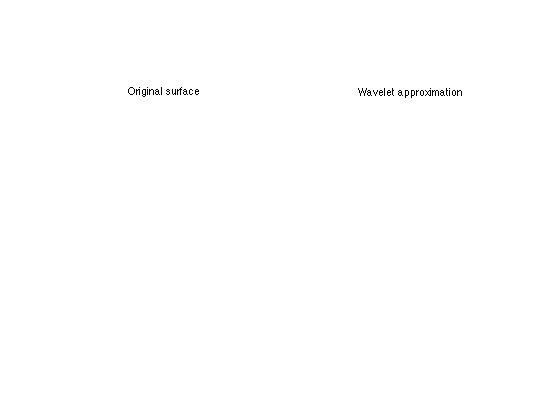$\newcommand{\NN}{\mathbb{N}} \newcommand{\CC}{\mathbb{C}} \newcommand{\GG}{\mathbb{G}} \newcommand{\LL}{\mathbb{L}} \newcommand{\PP}{\mathbb{P}} \newcommand{\QQ}{\mathbb{Q}} \newcommand{\RR}{\mathbb{R}} \newcommand{\VV}{\mathbb{V}} \newcommand{\ZZ}{\mathbb{Z}} \newcommand{\FF}{\mathbb{F}} \newcommand{\KK}{\mathbb{K}} \newcommand{\UU}{\mathbb{U}} \newcommand{\EE}{\mathbb{E}} \newcommand{\Aa}{\mathcal{A}} \newcommand{\Bb}{\mathcal{B}} \newcommand{\Cc}{\mathcal{C}} \newcommand{\Dd}{\mathcal{D}} \newcommand{\Ee}{\mathcal{E}} \newcommand{\Ff}{\mathcal{F}} \newcommand{\Gg}{\mathcal{G}} \newcommand{\Hh}{\mathcal{H}} \newcommand{\Ii}{\mathcal{I}} \newcommand{\Jj}{\mathcal{J}} \newcommand{\Kk}{\mathcal{K}} \newcommand{\Ll}{\mathcal{L}} \newcommand{\Mm}{\mathcal{M}} \newcommand{\Nn}{\mathcal{N}} \newcommand{\Oo}{\mathcal{O}} \newcommand{\Pp}{\mathcal{P}} \newcommand{\Qq}{\mathcal{Q}} \newcommand{\Rr}{\mathcal{R}} \newcommand{\Ss}{\mathcal{S}} \newcommand{\Tt}{\mathcal{T}} \newcommand{\Uu}{\mathcal{U}} \newcommand{\Vv}{\mathcal{V}} \newcommand{\Ww}{\mathcal{W}} \newcommand{\Xx}{\mathcal{X}} \newcommand{\Yy}{\mathcal{Y}} \newcommand{\Zz}{\mathcal{Z}} \newcommand{\al}{\alpha} \newcommand{\la}{\lambda} \newcommand{\ga}{\gamma} \newcommand{\Ga}{\Gamma} \newcommand{\La}{\Lambda} \newcommand{\Si}{\Sigma} \newcommand{\si}{\sigma} \newcommand{\be}{\beta} \newcommand{\de}{\delta} \newcommand{\De}{\Delta} \renewcommand{\phi}{\varphi} \renewcommand{\th}{\theta} \newcommand{\om}{\omega} \newcommand{\Om}{\Omega} \renewcommand{\epsilon}{\varepsilon} \newcommand{\Calpha}{\mathrm{C}^\al} \newcommand{\Cbeta}{\mathrm{C}^\be} \newcommand{\Cal}{\text{C}^\al} \newcommand{\Cdeux}{\text{C}^{2}} \newcommand{\Cun}{\text{C}^{1}} \newcommand{\Calt}{\text{C}^{#1}} \newcommand{\lun}{\ell^1} \newcommand{\ldeux}{\ell^2} \newcommand{\linf}{\ell^\infty} \newcommand{\ldeuxj}{{\ldeux_j}} \newcommand{\Lun}{\text{\upshape L}^1} \newcommand{\Ldeux}{\text{\upshape L}^2} \newcommand{\Lp}{\text{\upshape L}^p} \newcommand{\Lq}{\text{\upshape L}^q} \newcommand{\Linf}{\text{\upshape L}^\infty} \newcommand{\lzero}{\ell^0} \newcommand{\lp}{\ell^p} \renewcommand{\d}{\ins{d}} \newcommand{\Grad}{\text{Grad}} \newcommand{\grad}{\text{grad}} \renewcommand{\div}{\text{div}} \newcommand{\diag}{\text{diag}} \newcommand{\pd}{ \frac{ \partial #1}{\partial #2} } \newcommand{\pdd}{ \frac{ \partial^2 #1}{\partial #2^2} } \newcommand{\dotp}{\langle #1,\,#2\rangle} \newcommand{\norm}{|\!| #1 |\!|} \newcommand{\normi}{\norm{#1}_{\infty}} \newcommand{\normu}{\norm{#1}_{1}} \newcommand{\normz}{\norm{#1}_{0}} \newcommand{\abs}{\vert #1 \vert} \newcommand{\argmin}{\text{argmin}} \newcommand{\argmax}{\text{argmax}} \newcommand{\uargmin}{\underset{#1}{\argmin}\;} \newcommand{\uargmax}{\underset{#1}{\argmax}\;} \newcommand{\umin}{\underset{#1}{\min}\;} \newcommand{\umax}{\underset{#1}{\max}\;} \newcommand{\pa}{\left( #1 \right)} \newcommand{\choice}{ \left\{ \begin{array}{l} #1 \end{array} \right. } \newcommand{\enscond}{ \left\{ #1 \;:\; #2 \right\} } \newcommand{\qandq}{ \quad \text{and} \quad } \newcommand{\qqandqq}{ \qquad \text{and} \qquad } \newcommand{\qifq}{ \quad \text{if} \quad } \newcommand{\qqifqq}{ \qquad \text{if} \qquad } \newcommand{\qwhereq}{ \quad \text{where} \quad } \newcommand{\qqwhereqq}{ \qquad \text{where} \qquad } \newcommand{\qwithq}{ \quad \text{with} \quad } \newcommand{\qqwithqq}{ \qquad \text{with} \qquad } \newcommand{\qforq}{ \quad \text{for} \quad } \newcommand{\qqforqq}{ \qquad \text{for} \qquad } \newcommand{\qqsinceqq}{ \qquad \text{since} \qquad } \newcommand{\qsinceq}{ \quad \text{since} \quad } \newcommand{\qarrq}{\quad\Longrightarrow\quad} \newcommand{\qqarrqq}{\quad\Longrightarrow\quad} \newcommand{\qiffq}{\quad\Longleftrightarrow\quad} \newcommand{\qqiffqq}{\qquad\Longleftrightarrow\qquad} \newcommand{\qsubjq}{ \quad \text{subject to} \quad } \newcommand{\qqsubjqq}{ \qquad \text{subject to} \qquad }$

Wavelet Transform on 3D Meshes

# Wavelet Transform on 3D Meshes

This tour explores multiscale computation on 3D meshes using the lifting wavelet transform.

## Installing toolboxes and setting up the path.

You need to download the following files: signal toolbox, general toolbox, graph toolbox and wavelet_meshes toolbox.

You need to unzip these toolboxes in your working directory, so that you have toolbox_signal, toolbox_general, toolbox_graph and toolbox_wavelet_meshes in your directory.

For Scilab user: you must replace the Matlab comment '%' by its Scilab counterpart '//'.

Recommandation: You should create a text file named for instance numericaltour.sce (in Scilab) or numericaltour.m (in Matlab) to write all the Scilab/Matlab command you want to execute. Then, simply run exec('numericaltour.sce'); (in Scilab) or numericaltour; (in Matlab) to run the commands.

Execute this line only if you are using Matlab.

getd = @(p)path(p,path); % scilab users must *not* execute this


Then you can add the toolboxes to the path.

getd('toolbox_signal/');
getd('toolbox_general/');
getd('toolbox_graph/');
getd('toolbox_wavelet_meshes/');


## Functions Defined on Surfaces

One can define a function on a discrete 3D mesh that assigns a value to each vertex. One can then perform processing of the function according to the geometry of the surface. Here we use a simple sphere.

First compute a multiresolution sphere.

options.base_mesh = 'ico';
options.relaxation = 1;
options.keep_subdivision = 1;
J = 6;
[vertex,face] = compute_semiregular_sphere(J,options);


Options for the display.

options.use_color = 1;
options.rho = .3;
options.color = 'rescale';
options.use_elevation = 0;


Then define a function on the sphere. Here the function is loaded from an image of the earth.

f = load_spherical_function('earth', vertex{end}, options);


Display the function.

clf;
plot_spherical_function(vertex,face,f, options);
colormap gray(256);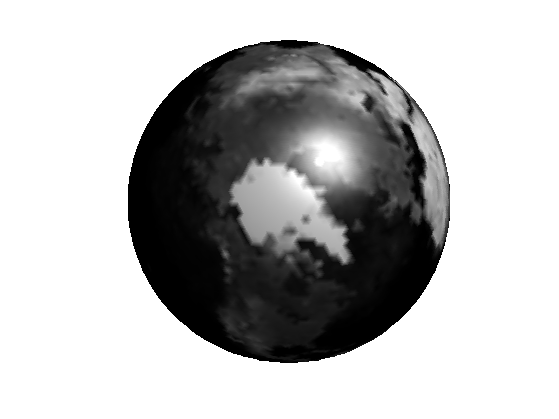## Wavelet Transform of Functions Defined on Surfaces

A wavelet transform can be used to compress a function defined on a surface. Here we take the example of a 3D sphere. The wavelet transform is implemented with the Lifting Scheme of Sweldens, extended to triangulated meshes by Sweldens and Schroder in a SIGGRAPH 1995 paper.

Perform the wavelet transform.

fw = perform_wavelet_mesh_transform(vertex,face, f, +1, options);


Threshold (remove) most of the coefficient.

r = .1;
fwT = perform_thresholding( fw, round(r*length(fw)), 'largest' );


Backward transform.

f1 = perform_wavelet_mesh_transform(vertex,face, fwT, -1, options);


Display it.

clf;
subplot(1,2,1);
plot_spherical_function(vertex,face,f, options);
title('Original function');
subplot(1,2,2);
plot_spherical_function(vertex,face,f1, options);
title('Approximated function');
colormap gray(256);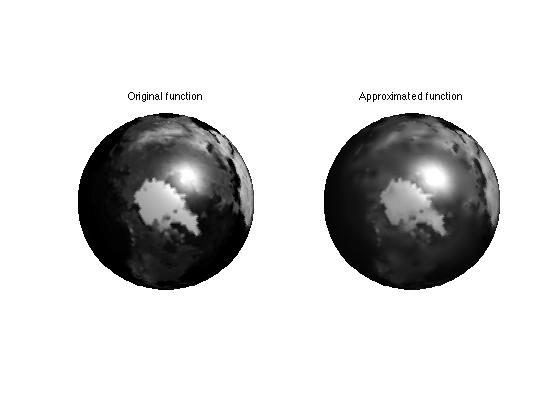Exercice 1: (check the solution) Plot the approximation curve error as a function of the number of coefficient.

exo1;


Exercice 2: (check the solution) Perform denoising of spherical function by thresholding. Study the evolution of the optimal threshold as a function of the noise level.

exo2;


Exercice 3: (check the solution) Display a dual wavelet that is used for the reconstruction by taking the inverse transform of a dirac.

exo3;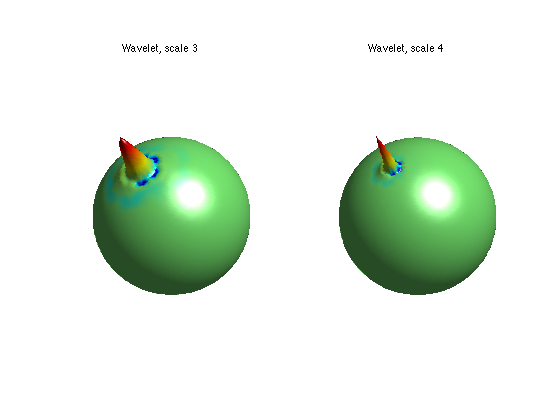## Spherical Geometry Images

A simple way to store a mesh is using a geometry images. This will be usefull to create a semi-regular mesh.

Firs we load a geometry image, which is a (n,n,3) array M where each M(:,:,i) encode a X,Y or Z component of the surface. The concept of geometry images was introduced by Hoppe and collaborators.

name = 'bunny';
n = size(M,1);


A geometry image can be displayed as a color image.

clf;
imageplot(M);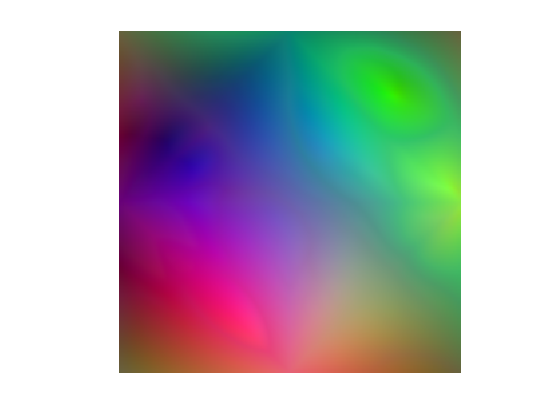But it can be displayed as a surface. The red curves are the seams in the surface to map it onto a sphere.

clf;
plot_geometry_image(M, 1,1);
view(20,88);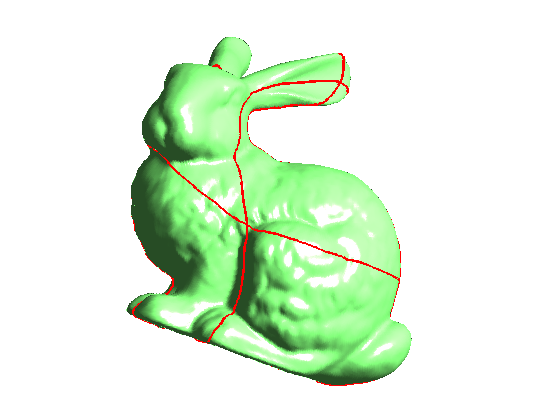One can compute the normal to the surface, which is the cross product of the tangent.

Compute the tangents.

options.order = 2;
u = zeros(n,n,3); v = zeros(n,n,3);
for i=1:3
end


Compute normal.

v = cat(3, u(:,:,2).*v(:,:,3)-u(:,:,3).*v(:,:,2), ...
u(:,:,3).*v(:,:,1)-u(:,:,1).*v(:,:,3), ...
u(:,:,1).*v(:,:,2)-u(:,:,2).*v(:,:,1) );


Compute lighting with an inner product with the lighting vector.

L = [1 2 -1]; L = reshape(L/norm(L), [1 1 3]);
A1 = max( sum( v .* repmat(L, [n n]), 3 ), 0 );
L = [-1 -2 -1]; L = reshape(L/norm(L), [1 1 3]);
A2 = max( sum( v .* repmat(L, [n n]), 3 ), 0 );


Display.

clf;
imageplot(A1, '', 1,2,1);
imageplot(A2, '', 1,2,2);## Semi-regular Meshes

To be able to perform computation on arbitrary mesh, this surface mesh should be represented as a semi-regular mesh, which is obtained by regular 1:4 subdivision of a base mesh.

Create the semi regular mesh from the Spherical GIM.

J = 6;
[vertex,face,vertex0] = compute_semiregular_gim(M,J,options);


Options for display.

options.func = 'mesh';
options.name = name;
options.use_elevation = 0;
options.use_color = 0;


We can display the semi-regular mesh.

selj = J-3:J;
clf;
for j=1:length(selj)
subplot(2,2,j);
plot_mesh(vertex{selj(j)},face{selj(j)}, options);
% title(['Subdivision level ' num2str(selj(j))]);
end
colormap gray(256);## Wavelet Transform of a Surface

A wavelet transform can be used to compress a suface itself. The surface is viewed as a 3 independent functions (X,Y,Z coordinates) and there are three wavelet coefficients per vertex of the mesh.

The function to process, the positions of the vertices.

f = vertex{end}';


Forward wavelet tranform.

fw = perform_wavelet_mesh_transform(vertex,face, f, +1, options);


Threshold (remove) most of the coefficient.

r = .1;
fwT = perform_thresholding( fw, round(r*length(fw)), 'largest' );


Backward transform.

f1 = perform_wavelet_mesh_transform(vertex,face, fwT, -1, options);


Display the approximated surface.

clf;
subplot(1,2,1);